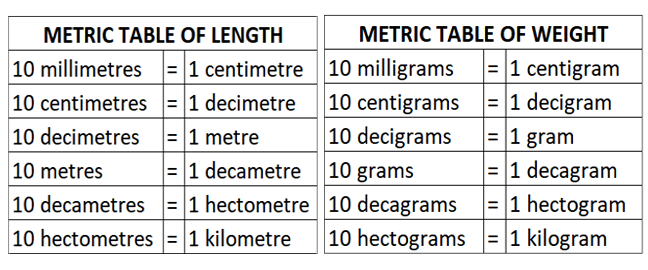# Equivalents of Units of Measurement

### *** इस पृष्ठ को हिंदी में पढ़े ***‌

Units of Length
1 inch = 2.54 centimetres1 cm = 0.3938 inches
1 yard = 0.9144 metres1 metre = 1.093 yards
1 mile = 1.609 km1 kilometre = 0.6213 miles
1 nautical mile = 1.852 kilometres1 kilometre = 0.5399 nautical miles
1 fathom (6 feet) = 1.828 metres1 metre = 0.5468 fathoms
1 mile = 5280 feet1 nautical mile = 6076.16 feet

Units of Weight
1 ounce = 28.349 grams1 gram = 0.03527 ounces
1 pound = 453.592 grams1 kilogram = 2.204 pounds
1 bale (cotton in India) = 170 kilograms

Units of Volume
1 gallon = 3.785 litres1 litre = 0.264 gallons
1 litre = 1000 cubic centimetres1 cc = 0.001 litre
1 litre = 2.1134 pints1 pint = 0.4731 litre
1 barrel (oil) = 158.99 litres1 barrel = 42 US gallons

Units of Area
1 acre = 4046.85 square metres1 square metre = 0.000247 acres
1 hectare = 10,000 square metres1 square metre = 0.0001 hectare

Units of Quantity
1 quire = 25 sheets1 ream = 500 sheets
1 bundle = 1,000 sheets1 bale = 5,000 sheets
1 dozen = 12 units1 gross = 12 dozen

### Metric Table of Length and Weight